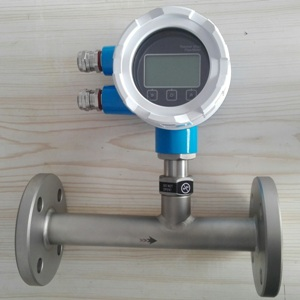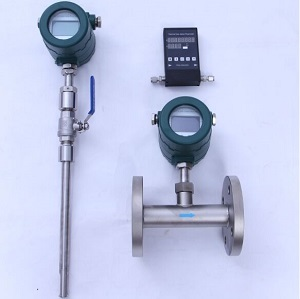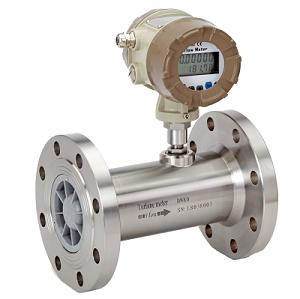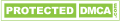# Negative Pressure Flow meter

• ### Thermal Mass Flow MeterChina thermal mass flow meter with cheap price. Gas flow meter for compressed air flow meter,biogas flow meter ,LPG flow...

• ### Gas Turbine Flow MeterRobust Gas flow meter for natural gas,LPG,biogas flow measurement.Inquiry Gas TUF Flowmeter price with EVC directly from...

## Negative Pressure Flow meter

Thermal mass flow meter can work on negative pressure in the pipeline, when flow meter is installed at the suction side of the super charger or pump, the pressure inside the pipeline could be negative pressure such as -10mbar or even cause cavitations, however thermal mass flow meter can work at that negative pressure environment.

## What is thermal mss flow meter?

A thermal mass flow meter is a type of flow meter that measures the flow rate of a fluid (usually a gas or air) by measuring the amount of heat that is transferred from a heated object to the fluid. The basic principle behind a thermal mass flow meter is that as gas or air flows past a heated object, the amount of heat that is transferred from the object to the fluid depends on the mass flow rate of the fluid.
A thermal mass flow meter typically consists of a sensor that contains a heated object (usually a platinum resistance temperature detector or RTD) and a temperature sensor. The heated object is maintained at a constant temperature, and as fluid flows past it, the amount of heat that is transferred to the fluid is detected by the temperature sensor. By measuring the temperature difference between the heated object and the fluid, the flow rate of the fluid can be calculated. The mass flow measurement result is independent of pressure, and negative pressure pipeline can place thermal flow meter to do the measurement.
Thermal mass flow meters are commonly used in industrial applications where accurate measurement of gas flow rates is required, such as in natural gas pipelines, chemical plants, and power plants. They are particularly useful for measuring low flow rates and for measuring the flow rate of gases with varying compositions or densities or even under negative pressure condition.

## Gas can be measured by thermal mass flow meter or negative pressure flow meter

Thermal mass flow meters can measure a wide variety of gases, including but not limited to:
• Thermal mass Air flowmeter
• Nitrogen mass flow meter
• Negative pressure air flow measurement
• Oxygen flow sensor
• Hydrogen mass flow transmitter
• Helium mass flow meter
• Thermal mass flow meter for methane flow measurement
• Carbon Dioxide (CO2) low price mass flow meter
• Natural Gas thermal mass flow meter;
• Propane mass flow meter
• Ethylene flow meter using thermal diffusion mass flow meter
• Butane mass flow meter
• Ammonia flow meter
• Biogas flow meter
It's important to note that different thermal mass flow meter models may have different measurement ranges and accuracy specifications for each gas, so it's important to choose a model that is specifically designed for the gas you wish to measure. Additionally, some thermal mass flow meters may be more suitable for certain gas measurement applications than others. For example you should inform us whether your gas or air at negative pressure or high pressure condition, your gas is corrosive or not? It is hot temperature or normal temperature? Therefore, it's important to consult with a thermal mass flow meter factory to determine which thermal mass flow meter is best for your particular application.

Gas turbine flow meter can also work on negative pressure, and in some gas turbine flow meter factory, the flow meter is calibrated under negative pressure. However vortex flow meter is not recommended to work under negative pressure.